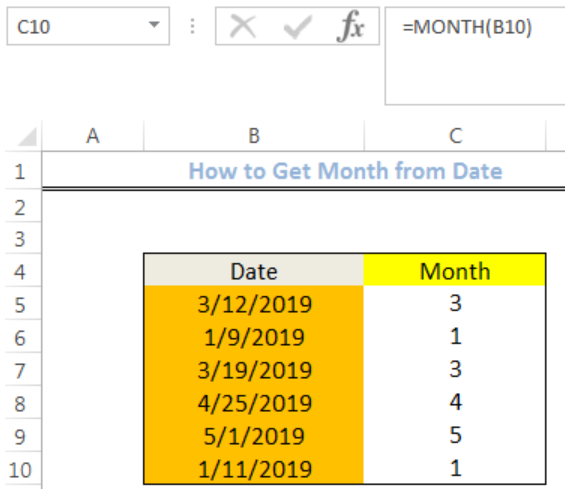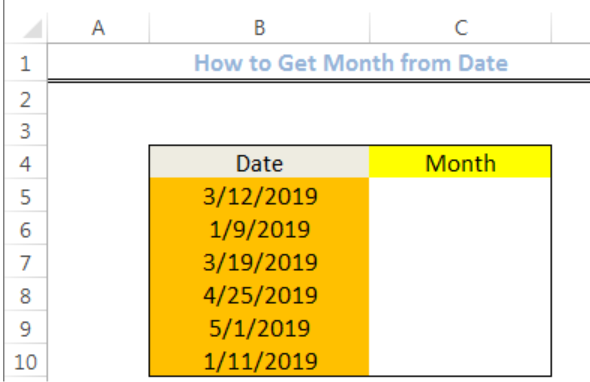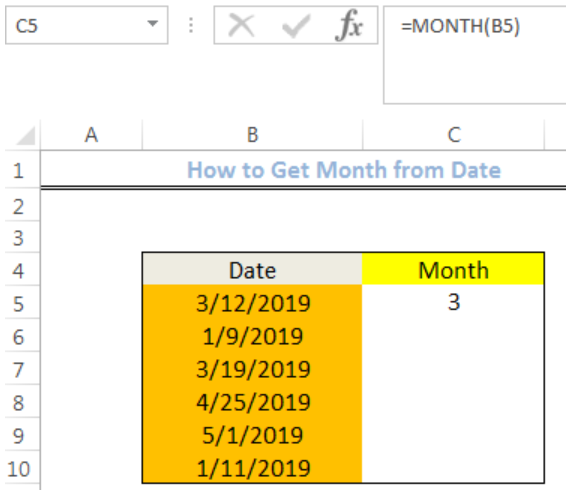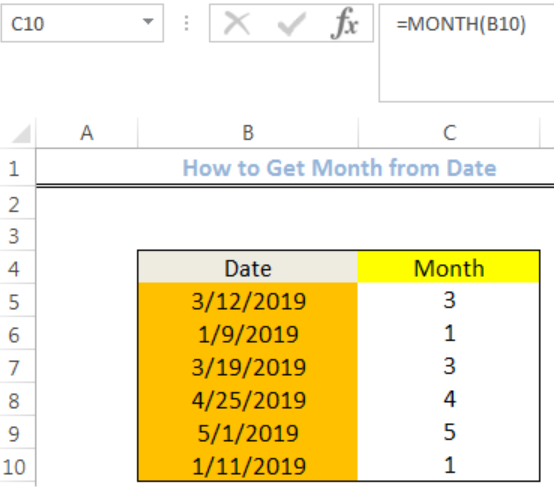Get instant live expert help with Excel or Google Sheets“My Excelchat expert helped me in less than 20 minutes, saving me what would have been 5 hours of work!”

#### Post your problem and you’ll get expert help in seconds.

Your message must be at least 40 characters
Our professional experts are available now. Your privacy is guaranteed.

# Get month from date

We can use the MONTH function to get the month from a given date. The steps below will walk through the process.Figure 1: Result of using the MONTH function

## Syntax

`=MONTH(date)`

## Formula

`=MONTH(B5)`

## Setting up the Data

• We will set up data by inputting our Dates in Column B
• Our result, which is the month, will be returned in Column CFigure 2: Setting up the Data

## Get the MONTH with the MONTH Function

• We will click on Cell C5
• We will insert the formula below into the cell
`=MONTH(B5)`
• We will press the enter keyFigure 3: How to get the month from date

• We will click on Cell C5 again
• We will double click on the fill handle tool which is the small plus sign you see at the bottom right of Cell C5. Select and drag down to copy the formula to other cells.Figure 4: Result of using the MONTH function

## Instant Connection to an Expert through our Excelchat Service

Most of the time, the problem you will need to solve will be more complex than a simple application of a formula or function. If you want to save hours of research and frustration, try our live Excelchat service! Our Excel Experts are available 24/7 to answer any Excel question you may have. We guarantee a connection within 30 seconds and a customized solution within 20 minutes.

### Did this post not answer your question? Get a solution from connecting with the expert.Another blog reader asked this question today on Excelchat:
Solution exampleshow do I derive the monthly sales from a date and revenue column
Solved by A. U. in 23 minsI can't get this formula correct, can someone assist? I am trying to create a date for our posting process based on the payment terms. If a customer is paying biweekly, with a start date of 3/1/2018, etc. I want to be able to run daily what customers will need to be charged. =IF(OR(D2="MONTHLY",\$C\$6+30,")*IF(D2="WEEKLY",\$C\$6+7,"")*IF(D2="BIWEEKLY",\$C\$6+14,""""))
Solved by S. H. in 9 minsHOW CAN I COUNT NUMBERS PER DAY FOR A MONTH AS TOTAL?
Solved by F. F. in 16 minsa date formula that results in showing the date as 29/12/00 (date format as dd/mm/yy) - use column BA to calculate 364 days (same date format) in column BS, if BA blank, then calculate 364 days using column AX. Results for column BA are correct. However, if column BA is empty, then it should calculate using column AX, which has data, but the result is always 29/12/00, regardless of the date in column AX. I have used this formula with success in another workbook, but this file wont work! Formula: =IF(AND(LEN(\$BA74)=0,LEN(\$AX74)=0),"",IF(LEN(\$BA74)=0,DATE(YEAR(\$AX74),MONTH(\$AX74),DAY(\$AX74)+364),DATE(YEAR(\$BA74),MONTH(\$BA74),DAY(\$BA74)+364)))
Solved by A. L. in 60 minsI need to sum the number of hours per month one of three halls are being rented
Solved by A. F. in 60 mins## Subscribe to Excelchat.coAnother blog reader asked this question today on Excelchat: